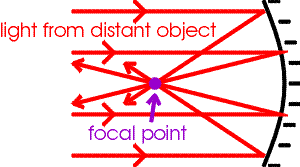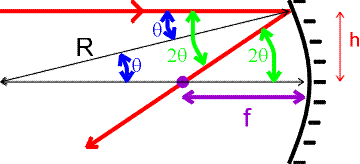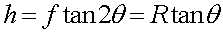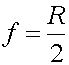Focal points of curved mirrorsMirrors can focus light, and can therefore be used to create images in a manner that is similar to that of lenses which will be discussed later.

It will be helpful to keep in mind three prototypical examples. The primary example (for which the "image distance" will be positive and the image will be "real"), is the telescope mirror. A telescope mirror is a concave mirror such as a satelite dish or the Hubble space telescope, that is used to form a real image of a far-away object. (We will find that "far away" means farther away than the focal point.) The second prototypical example is the shaving/beauty mirror which is also a concave mirror, but which is used to form an enlarged virtual image of an object that is closer to the mirror than the focal length. The third prototypical example is the garden ball, which is a convex mirror and forms a virtual image that is smaller than the object. (The curved side-view mirror in an automobile is also an example of this type; they generally carry a warning that "objects are closer than they appear," because the image of the semi behind you is smaller than the truck itself.)

To understand focusing, first consider light rays from an infinitely distant object that arrive at a concave mirror. The sketch shows how light from the object that hits the mirror is focused to a single point called the focal point.To demonstrate that all lines regardless of h are focused to a single point, consider a spherical mirror with a radius of curvature R. Light that hits the surface an angle q from the normal will reflect off at an angle of 2q from the normal. Some trigonometry will now give the focal length in terms of R.For small angles, tanq approximately equals q and tan2q approximately equals 2q, thusThe fact that this crossing point is independent of h shows that all horizontal rays will be reflected through the same point. (We have assumed the mirror is spherical and that the angle is small; a parabolic mirror would focus light to the same point exactly, even for large angles).

Aside from telescopes, focusing like this is used in solar collectors to bring focus sunlight to single point to be converted into electrical energy. Similar focussing is done for radio waves by a satelite dish.

Mirrors index      examples        Lecture index